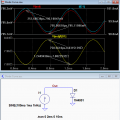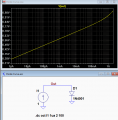# Diode in series with Resistor vs Diode and its inherent resistance

#### Clueless123

Joined Jan 5, 2019
6
Hello,

Could someone explain to me how the inherent resistance in a diode would NOT function the same way as a diode connected in series with a resistor?

#### BobTPH

Joined Jun 5, 2013
3,820
The diode does not have an inherent resistance. The ratio of I to V is exponential vs linear for a resistor.

Bob

#### Clueless123

Joined Jan 5, 2019
6
The diode does not have an inherent resistance. The ratio fo I to V is exponential vs linear for a resistor.

Bob
my apologies. in some simulation software, the diode is modeled as an ideal diode in series with an offset voltage source and a resistor. am i correct in that the person who argued the above has mistaken the resistor used simply to show the non-ideal characteristics the diode to be an actual resistor? thanks.

#### Clueless123

Joined Jan 5, 2019
6
The diode does not have an inherent resistance. The ratio of I to V is exponential vs linear for a resistor.

Bob
Noting that the small-signal resistance {\displaystyle r_{D}}is the reciprocal of the small-signal conductance just found, the diode resistance is independent of the ac current, but depends on the dc current, and is given as

{\displaystyle r_{D}={\frac {n\cdot V_{\text{T}}}{I_{Q}}}}.

https://en.wikipedia.org/wiki/Diode_modelling

is this wrong then???

Joined Mar 10, 2018
4,057

#### crutschow

Joined Mar 14, 2008
27,945
is this wrong then???
No.
The diode does have a small-signal apparent impedance, which basically is the linearization of a small portion of the log curve of current versus voltage.
This approximation is only valid for a small AC current (AC current << DC current).

For example, the LTspice simulation below shows the AC impedance for an AC current that is 1% of the DC current
The voltage variation (red trace) is about 1 mVpp for a 2 mApp change in current (blue trace), giving an AC impedance of about 1/2 ohm.
The apparent DC impedance change (yellow trace) is about 1.9%, which indicates how much the AC impedance changes over the ACpp current change.Diodes also have a small parasitic resistance in series the ideal diode characteristics, which becomes more apparent at high current levels.
This is shown in the simulation below where the straight line log curve of voltage vs. current starts curving upward above about 0.2A for a 1N4001 diode.#### Clueless123

Joined Jan 5, 2019
6

i realize that a diode may have a resistance but would it be correct to say that a typical circuit designer would not treat a diode in series with a resistor as the same as a diode with its inherent (forward and reverse) resistance? for one thing, the voltage drop (threshold voltage) across a diode is typical constant at 0.7V ish regardless of current, whereas for a resistor, the voltage drop varies linearly with the current.

i am really looking for evidence, preferably from academic or trusted sources, that verify my position that a typical circuit designer would not view a diode in series with a resistor to be an obvious design variation for a mere diode, particularly for a commutation cell as shown in the figures i uploaded. (ie. in the first figure, diode Don and resistor Ron are not obvious given the second circuit with just a diode D3). Thanks!

#### crutschow

Joined Mar 14, 2008
27,945
the voltage drop (threshold voltage) across a diode is typical constant at 0.7V ish regardless of current,
No.
Look at my second simulation.
The voltage is around 0.7V for a current of 20mA, but it's about 0.6V @ 1mA and 0.8V @ 100mA.

#### Clueless123

Joined Jan 5, 2019
6
No.
Look at my second simulation.
The voltage is around 0.7V for a current of 20mA, but it's about 0.6V @ 1mA and 0.8V @ 100mA.
i realize there are minor variations in voltage but for the purposes of designing a circuit such as a commutation cell to be used with a IGBT switch for limiting switching loss, the small signal resistance and variations in milli-amps would not impact your design decisions right?a typical designer would not use the model to the left to mean that there is an actual resistor in the circuit, but understand that this circuit is used for simulation purposes where the resistor is used to approximate the exponential relationship in the diode I-V graph.

#### crutschow

Joined Mar 14, 2008
27,945
the small signal resistance and variations in milli-amps would not impact your design decisions right?
Yes, as long as the circuit is not sensitive to a few tenths of a volt change in the diode forward drop.
a typical designer would not use the model to the left to mean that there is an actual resistor in the circuit, but understand that this circuit is used for simulation purposes where the resistor is used to approximate the exponential relationship in the diode I-V graph.
The resistor is used to simulate the parasitic diode resistance, not the exponential relationship of current to voltage.
To simulate the exponential relationship you need a voltage source whose voltage is exponentially proportional to current.
Most designers use Spice simulators which have such an exponential model available in the model files.

•Clueless123